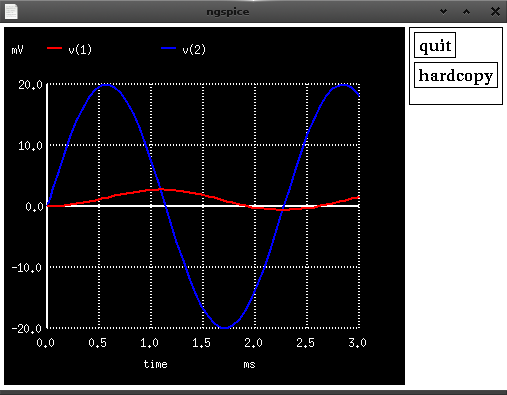# shorne in japan

## Spice Essentials

21 Apr 2015

Did you ever get stuck figuring out how to run spice? These essential spice tips will get you on your way.

Recently I have been searching for good linux tools to simulate circuits. Spice is widely known in circuit simulation. This short guide should help you get started; including how to perform graphical plotting with nutmeg. All the below demos are using `ngspice` and `ngnutmeg`. In this post I assume you know the basics of writing or generating spice netlists.

We will cover the basics of:

• Analysis with `OP` and `TRAN`
• Controlling spice output
• Running `ngspice` and `ngnutmeg`

## Analysis

When analyzing a circuit we need to use spice analysis commands. These are usually placed at the end of your netlist.

### Using OP

The first and most simple analysis command you should know is the `OP` command. It provides the dc operating point voltage dump of all nodes with capacitors fully charged (no current) and inductors fully inducting (shorted).

Usage

``````.op
``````

Example

``````* OP analysis of Voltage divider
V1 2 0 DC 10V
R1  2 1 50K
R2  1 0 20K
.op
.end
``````

When running we can see that the voltage and current of each node is displayed.

``````\$ ngspice -b op.cir

No. of Data Rows : 1
Node                                  Voltage
----                                  -------
----    -------
V(1)                             2.857143e+00
V(2)                             1.000000e+01

Source  Current
------  -------

v1#branch                        -1.42857e-04
``````

### Using TRAN

The next analysis command to know is the `TRAN` command.

`TRAN` will run the circuit for a fixed time and take interval measurements. The below `.tran 0.1m 5m` will measure for 5 milliseconds and output every 0.1 milliseconds. It should print 50 readings. You should choose your measurements based on the frequency of the input source to allow you to see the full wave,

Usage

`.tran STEP END <START> <MAX>`

• STEP - is the time interval of how often a measurement is taken
• END - is the time when spice will end measurement
• START - (default 0) is the time when spice will start measurement
• MAX - is used to define a STEP smaller than STEP (yeah confusing)

Note: spice manuals mention the step time of `TRAN` is not always used by spice. If `END-START/50` is less than `STEP` spice will set step to the smaller value. I haven’t seen this affect my simulations yet.

Example

``````* TRAN analysis of RC circuit
Vin  2 0 AC SIN(0 0.02 440)
R5  2 1 1K
C1  1 0 4.7uF
.tran 0.1m 3m
.plot tran v(2), v(1)
.end
``````

When running we can see a plot printed of our analysis.

``````\$ ngspice -b tran.cir

No. of Data Rows : 63
--------------------------------------------------------------------------
* ac analysis of rc circuit
Transient Analysis Tue Apr 21 22:55:08  2015

Legend:  + = v(2)             * = v(1)
--------------------------------------------------------------------------
time       v(2)    -2.00e-02   -1.00e-02    0.00e+00    1.00e-02    2.00e-02
----------------------|-----------|-----------|-----------|-----------|
0.000e+00  0.000e+00 .           .           X           .           .
1.000e-04  5.456e-03 .           .           *     +     .           .
2.000e-04  1.047e-02 .           .           *           +           .
3.000e-04  1.471e-02 .           .           *           .    +      .
4.000e-04  1.786e-02 .           .           *           .        +  .
5.000e-04  1.958e-02 .           .           .*          .          +.
6.000e-04  1.987e-02 .           .           .*          .          +.
7.000e-04  1.867e-02 .           .           . *         .         + .
8.000e-04  1.598e-02 .           .           . *         .      +    .
9.000e-04  1.213e-02 .           .           .  *        . +         .
1.000e-03  7.356e-03 .           .           .  *    +   .           .
1.100e-03  2.000e-03 .           .           . +*        .           .
1.200e-03 -3.490e-03 .           .      +    .  *        .           .
1.300e-03 -8.736e-03 .           .+          . *         .           .
1.400e-03 -1.328e-02 .       +   .           . *         .           .
1.500e-03 -1.684e-02 .  +        .           . *         .           .
1.600e-03 -1.916e-02 .+          .           .*          .           .
1.700e-03 -1.993e-02 +           .           .*          .           .
1.800e-03 -1.926e-02 +           .           *           .           .
1.900e-03 -1.714e-02 .  +        .           *           .           .
2.000e-03 -1.365e-02 .      +    .          *.           .           .
2.100e-03 -9.168e-03 .           +          *.           .           .
2.200e-03 -3.990e-03 .           .      +   *.           .           .
2.300e-03  1.502e-03 .           .          *.+          .           .
2.400e-03  6.873e-03 .           .          *.       +   .           .
2.500e-03  1.175e-02 .           .          *.           . +         .
2.600e-03  1.567e-02 .           .           *           .     +     .
2.700e-03  1.845e-02 .           .           *           .         + .
2.800e-03  1.986e-02 .           .           *           .          +.
2.900e-03  1.967e-02 .           .           .*          .          +.
3.000e-03  1.810e-02 .           .           .*          .        +  .
----------------------|-----------|-----------|-----------|-----------|
time       v(2)    -2.00e-02   -1.00e-02    0.00e+00    1.00e-02    2.00e-02

elapsed time since last call: 0.018 seconds.
``````

## Controlling Output

To output data during simulation we use the `PLOT` and `PRINT` commands. These commands work together with dynamic analysis commands like `TRAN` to output analysis data points.

The `plot` command will plot a ascii chart of analysis.

``````.plot tran v(1), v(8)
``````

The `print` command will print the value of the analysis.

``````.print tran v(1), v(8)
``````

## Running Spice

When simulating a circuit we have a few options.

``````# Running in batch mode
\$ ngspice -b op.cir
``````
``````# Running in interactive move
\$ ngspice
> source op.cir
> run
# will display output as above
> edit
# Will allow editing of your circuit
``````

Another option is to output the analysis data in raw format. This data can then be loaded up in ngnutmeg for further analysis.

``````# This commands runs the analysis in preamp.cir and outputs raw data to
# tran.raw
ngspice -b tran.cir -r tran.raw
``````
``````# The below command loads the raw file into ngnutmeg and then displays the
# ngnutmeg prompt. The `plot v(1)` command will bring up a view of the wave form
ngnutmeg tran.raw
> plot v(2) v(1)
``````